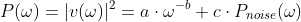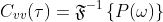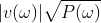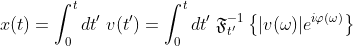## Friday, April 4, 2008

### [AD 1] The "Noise+PL_Cvv" Model

Question:

Are Carina's data compatible with the simple assumption of strictly powerlaw-correlated velocity fluctuations on a white noise floor, or do we need extra model ingredients ?

Ansatz:

On a discrete time-/frequency grid, we generate a power spectral density (PSD) according to above assumption:According to the Wiener-Kinchin theorem, P(w) is the Fourier transform of the velocity auto-correlation function (VAC):The VAC in turn determines the mean squared displacement (MSD) of the particle:In other words, the spectra PSD, VAC and MSD express the same information in different ways and are in the above model characterized by only three parameters a,b and c.

The square root of P(w) gives the frequency-dependent modulus of the velocity oscillations:If we multiply |v(w)| by an arbitrary, frequency-dependent phase function, this will leave the PSD, VAC and MSD invariant:But this operation will have a drastic effect on the shape of the resulting trajectory in real space and on other properties which are independent from the MSD:In other words, we can explore a huge ensemble of different trajectories (by chosing for example random phases at each discrete frequency), which however all produce the very same MSD.

We can then find out if this space of trajectories is sufficiently rich to reproduce all the different signatures found in the data.